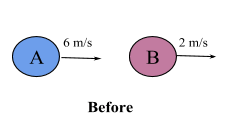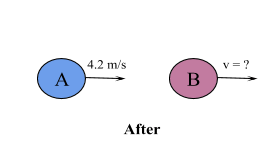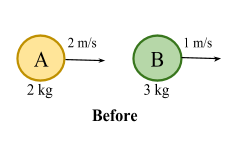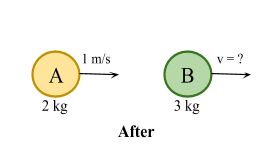# Impulse and Momentum

Contents

## Summary

• Momentum is a measure of the quantity of motion of a moving body, due to its mass and velocity. ( Momentum = Mass X Velocity ).
• The law of conservation of momentum states total momentum before a collision equals total momentum after a collision.
• Impulse is basically change in momentum of a particle in the direction of motion.
• Impulse = mv – mu & Impulse = Force X Time

To understand what impulse is we first discuss momentum.

#### What is Momentum?

Momentum is a measure of quantity of motion of a moving body, due to its mass and velocity. In other words it is the measure of how much stuff is moving and how fast the stuff is moving.

It can be described using the formula:

Momentum = Mass X Velocity

The equation above illustrates that momentum is directly proportional to an object’s mass and directly proportional to the object’s velocity.

As momentum is a vector quantity, it does have a direction and hence the sign of the velocity is important. We can say that the direction of the momentum vector is same as the direction of the velocity vector. Also the unit of momentum is$kgm{ s }^{ -1 }$.

Momentum is usually denoted by symbol the$''\rho''$  and hence the above equation can also be written as:$\rho \quad =\quad m\quad \times \quad v$

Let’s move on to discuss the concept of ”conservation of momentum”. Conservation of momentum basically is that total momentum before a collision equals total momentum after a collision.

#### Example #1

Q. Particles A and B, each of mass 5 kg, move in a straight line with velocities 6$m{ s }^{ -1 }$ and 2$m{ s }^{ -1 }$ respectively. After collision mass A continues in the same direction with velocity 4.2$m{ s }^{ -1 }$. Find the velocity of particle B after impact.

Solution:As we know that the law of conservation of momentum states total momentum before a collision equals total momentum after a collision.

Therefore:

Momentum of A + Momentum of B = Momentum of A + Momentum of B$\Rightarrow \quad equation1$

We can write:

mass of A is${ m }_{ 1 }\quad =\quad 5\quad kg$

velocity of A before collision${ u }_{ 1 }\quad =\quad 6\quad m{ s }^{ -1 }$

mass of B is${ m }_{ 2 }\quad =\quad 5\quad kg$

velocity of B before collision${ u }_{ 2 }\quad =\quad 2\quad m{ s }^{ -1 }$

velocity of A after collision${ v }_{ 1 }\quad =\quad 4.2\quad m{ s }^{ -1 }$

velocity of A after collision${ v }_{ 2 }\quad =\quad ?$

Substitute the above values in equation 1:${ m }_{ 1 }{ u }_{ 1 }\quad +\quad { m }_{ 2 }{ u }_{ 2 }\quad =\quad { m }_{ 1 }{ v }_{ 1 }\quad +\quad { m }_{ 2 }{ v }_{ 2 }$$5(6)\quad +\quad 5(2)\quad =\quad 5(4.2)\quad +\quad 5{ v }_{ 2 }$$40\quad =\quad 21\quad +\quad 5{ v }_{ 2 }$${ v }_{ 2 }\quad =\quad 3.8\quad m{ s }^{ -1 }$    in the same direction as before      Ans

Note: If after collision particles join together, we can treat them as just one object and therefore they have the same velocity.

#### What is Impulse?

Impulse is basically change in momentum of a particle in the direction of motion. It quantifies the overall effect of a force acting over time on a particle.

To work out impulse we simply subtract the particles initial momentum from its final momentum. Impulse is measured in Ns.

Formula to work out impulse is:

Impulse = mv – mu
Where v is the final velocity and u is the initial velocity

As we have defined above that impulse is also related to the force needed to change the momentum and the time it takes.

Impulse = Force X Time

#### Example #2

Q. A mass of 2 kg moving at 2$m{ s }^{ -1 }$  collides with a mass of 3 kg which is moving in the same direction at 1$m{ s }^{ -1 }$. The 2 kg mass continues to move in the same direction at 1$m{ s }^{ -1 }$ after impact. Find the impulse given by the 2 kg mass to the other mass.

Solution:Using law of conservation of momentum:${ m }_{ 1 }{ u }_{ 1 }\quad +\quad { m }_{ 2 }{ u }_{ 2 }\quad =\quad { m }_{ 1 }{ v }_{ 1 }\quad +\quad { m }_{ 2 }{ v }_{ 2 }$$2(2)\quad +\quad 3(1)\quad =\quad 2(1)\quad +\quad 3{ v }_{ 2 }$${ v }_{ 2 }\quad =\quad \frac { 5 }{ 3 } m{ s }^{ -1 }$

Now for impulse on B:

Impulse on$B\quad =\quad { m }_{ 2 }{ v }_{ 2 }\quad -\quad { m }_{ 2 }{ u }_{ 2 }$$=\quad (3\quad \times \quad \frac { 5 }{ 3 } )\quad -\quad (3\quad \times \quad 1)$

Impulse on B = 2 Ns      Ans

##### Reference
1. CGP complete revision and practice guide AS- Level Mathematics Edexcel
2. https://www.physicsclassroom.com/Class/momentum/u4l1a.cfm